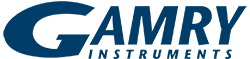# NERNST Calculator

The Nernst equation describes the relationship between electrode potential and solution concentration. In the equation below, Q is the reaction quotient and is the product of the activity times concentration for the reactants divided by the activity times concentration for the products for an overall reduction reaction. The Nernst equation is useful for predicting concentrations near the electrode surface at a given applied potential provided the cell responds quickly to changes in applied potential.

The potential of the working electrode determines what kind of redox processes may occur at the electrode's surface. Extremely negative potentials will probably reduce analytes while positive potentials are likely to oxidize them.

Below is our Nernst Equation Calculator. Here you are able to input E°, T, n, and Q (as O/R) and see the resulting Ecell.

### Calculate Ecell

×YES, WE ARE OPEN!

Gamry is accepting and shipping orders.  Our Technical Support and Sales are here to help.